﻿

### Upper semicontinuity of the spectrum function and automatic continuity in topological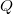$Q$-algebras

#### Abstract

In 1993, M. Fragoulopoulou applied thetechnique of Ransford and proved that if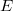$E$ and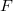$F$ are lmcalgebras such that$E$ is a Q-algebra,$F$ is semisimple andadvertibly complete, and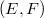$(E,F)$ is a closed graph pair, then eachsurjective homomorphism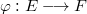$\varphi:E\longrightarrow F$ is continuous. Later onin 1996, it was shown by Akkar and Nacir that if$E$ and$F$ areboth LFQ-algebras and$F$ is semisimple then evey surjectivehomomorphism$\varphi:E\longrightarrow F$ is continuous. In this work weextend the above results by removing the lmc property from$E$.

We first show that in a topological algebra, the uppersemicontinuity of the spectrum function, the upper semicontinuityof the spectral radius function, the continuity of the spectralradius function at zero, and being a$Q$-algebra, are allequivalent. Then it is shown that if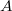$A$ is a topological$Q$-algebra and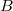$B$ is an lmc semisimple algebra which isadvertibly complete, then every surjective homomorphism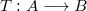$T:A\longrightarrow B$ has a closed graph. In particular, if$A$ is a Q-algebra with acomplete metrizable topology, and$B$ is a semisimple Fréchet algebra, then every surjective homomorphism$T:A\longrightarrow B$ isautomatically continuous.

DOI Code: 10.1285/i15900932v28n2p57

Keywords: automatic continuity; topological algebra; Fr´echet algebra; Q-algebra; spectrum function; spectral radius; upper semicontinuity; advertibly complete

Classification: 46H40; 46H05

Full Text: PDF